×#### Thank you for registering.

One of our academic counsellors will contact you within 1 working day.

Click to Chat

1800-1023-196

+91-120-4616500

CART 0

• 0

MY CART (5)

Use Coupon: CART20 and get 20% off on all online Study Material

ITEM
DETAILS
MRP
DISCOUNT
FINAL PRICE
Total Price: Rs.

There are no items in this cart.
Continue Shopping• Complete JEE Main/Advanced Course and Test Series
• OFFERED PRICE: Rs. 15,900
• View Details

```Chapter 11: Trigonometric Equations – Exercise 11.1

Trigonometric Equations – Exercise 11.1 – Q.1(i)

We have,Trigonometric Equations – Exercise 11.1 – Q.1(ii)

We have,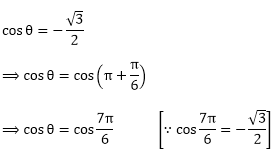∵  the general solution isTrigonometric Equations – Exercise 11.1 – Q.1(iii)

cosec θ = – √2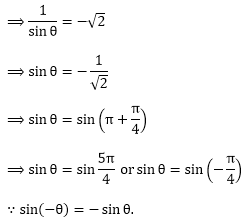∵ sin⁡(-θ) = -sin ⁡θ.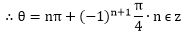Trigonometric Equations – Exercise 11.1 – Q.1(iv)

We have,Trigonometric Equations – Exercise 11.1 – Q.1(v)

We have,Trigonometric Equations – Exercise 11.1 – Q.1(vi)

We have,Trigonometric Equations – Exercise 11.1 – Q.2(i)

We have,Trigonometric Equations – Exercise 11.1 – Q.2(ii)

We have,Trigonometric Equations – Exercise 11.1 – Q.2(iii)

sin ⁡9θ = sin ⁡θ

sin ⁡9θ – sin ⁡θ = 0

Apply sin ⁡A sin ⁡B formulasin⁡ 9θ – sin ⁡θ = 2 cos⁡ 5θ sin ⁡4θ = 0

cos⁡ 5θ sin ⁡4θ = 0

⟹ cos ⁡5θ = 0(or)  sin ⁡4θ = 0Trigonometric Equations – Exercise 11.1 – Q.2(iv)

We have,

sin⁡ 2θ = cos⁡ 3θ

⟹ cos ⁡3θ = sin⁡2θ⟹ eitherTrigonometric Equations – Exercise 11.1 – Q.2(v)

We have,

tan⁡ θ + cot ⁡2θ = 0

tan ⁡θ = – cot ⁡2θ

⟹ cot ⁡2θ = – tan⁡θ

⟹ tan ⁡2θ = – cot⁡θTrigonometric Equations – Exercise 11.1 – Q.2(vi)

We have,

tan ⁡3θ = cot⁡θ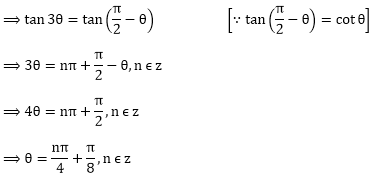Trigonometric Equations – Exercise 11.1 – Q.2(vii)

We have,

tan⁡ 2θ tan⁡θ = 1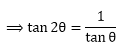⟹ tan ⁡2θ = cot⁡θTrigonometric Equations – Exercise 11.1 – Q.2(viii)

tan⁡ mθ + cot⁡ nθ = 0

sin⁡ mθ sin⁡ nθ + cos⁡ mθ  cos ⁡nθ = 0

cos⁡ (m – n)θ = 0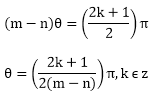Trigonometric Equations – Exercise 11.1 – Q.2(ix)

We have,

tan⁡ pθ = cot⁡qθ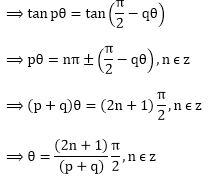Trigonometric Equations – Exercise 11.1 – Q.2(x)

sin ⁡2x + cos ⁡x = 0

2 sin⁡x cos⁡x + cos⁡x = 0

cos⁡x (2 sin⁡ +1) = 0

cos⁡x = 0 or 2 sin⁡x + 1 = 0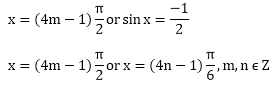Trigonometric Equations – Exercise 11.1 – Q.2(xi)

We have,

sin⁡ θ = tan ⁡θ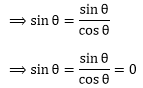⟹ sin⁡θ (cosθ – 1) = 0

⟹ either sin⁡θ = 0               or cos⁡θ – 1 = 0

⟹ θ = nπ, n ϵ z                    or cos⁡θ = 1

⟹ cos⁡θ = cos 0°

θ = 2mπ, m ϵ z

Thus,

θ = nπn ϵ z  or θ = 2mπ, m ϵ z

Trigonometric Equations – Exercise 11.1 – Q.2(xii)

cos⁡ (2x) = – sin⁡(3x)```### Course Features

• 728 Video Lectures
• Revision Notes
• Previous Year Papers
• Mind Map
• Study Planner
• NCERT Solutions
• Discussion Forum
• Test paper with Video Solution